# recreg

Recursive linear regression

## Syntax

[Coeff,SE] = recreg(X,y)
[CoeffTbl,SETbl] = recreg(Tbl)
___ = recreg(___,Name=Value)
recreg(___)
___ = recreg(ax,___)
[___,coeffPlots] = recreg(___)

## Description

recreg recursively estimates coefficients (β) and their standard errors in a multiple linear regression model of the form y = + ε by performing successive regressions using nested or rolling windows. recreg has options for OLS, HAC, and FGLS estimates, and for iterative plots of the estimates.

example

[Coeff,SE] = recreg(X,y) returns a matrix of regression coefficient estimates Coeff and a corresponding matrix of standard error estimates SE from recursive regressions of the multiple linear regression model y = Xβ + ε.

example

[CoeffTbl,SETbl] = recreg(Tbl) returns regression coefficients estimates in the table CoeffTbl, and standard error estimates in the table SETbl from a recursive regression on the linear model of the variables in the table or timetable Tbl.The response variable in the regression is the last table variable, and all other variables are the predictor variables. To select a different response variable for the regression, use the ResponseVariable name-value argument. To select different predictor variables, use the PredictorNames name-value argument.

example

___ = recreg(___,Name=Value) uses additional options specified by one or more name-value arguments, using any input-argument combination in the previous syntaxes. recreg returns the output-argument combination for the corresponding input arguments. For example, recreg(Tbl,ResponseVariable="GDP",Intercept=false,Estimator="fgls") excludes an intercept term from the regression model, in which the response variable is the variable GDP in the table Tbl, and uses FGLS to estimate coefficients and standard errors.

example

recreg(___) plots iterative coefficient estimates with ±2 standard error bands for each coefficient in the multiple linear regression model.
___ = recreg(ax,___) plots on the axes specified in ax instead of the axes of new figures. The option ax can precede any of the input argument combinations in the previous syntaxes.
[___,coeffPlots] = recreg(___) additionally returns handles to plotted graphics objects. Use elements of coeffPlots to modify properties of the plots after you create it.

## Examples

collapse all

Check coefficient estimates for instability in a model of food demand around World War II. Implement forward and backward recursive regressions in a rolling window.

Load the US food consumption data set, which contains annual measurements from 1927 through 1962 with missing data due to WWII.

load Data_Consumption

For more details on the data, enter Description at the command prompt.

Plot the series.

P = Data(:,1); % Food price index I = Data(:,2); % Disposable income index Q = Data(:,3); % Food consumption index figure plot(dates,[P I Q]) axis tight grid on xlabel("Year") ylabel("Index") title("\bf Time Series Plot of All Series") legend("Price","Income","Consumption",Location="southeast")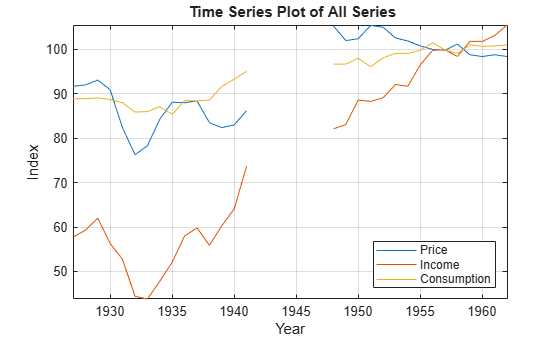Measurements are missing from 1942 through 1947, which correspond to WWII.

To examine elasticities, apply the log transformation to each series.

LP = log(P); LI = log(I); LQ = log(Q);

Consider a model in which log consumption is a linear function of the logs of food price and income. In other words,

${\text{LQ}}_{t}={\beta }_{0}+{\beta }_{1}{\text{LI}}_{t}+{\beta }_{2}\text{LP}+{\epsilon }_{t}.$

${\epsilon }_{t}$ is a Gaussian random variable with mean 0 and standard deviation ${\sigma }^{2}$.

Identify the breakpoint index at the end of WWII, 1945. Ignore missing years with missing data.

numCoeff = 4; % Three predictors and an intercept T = numel(dates(~isnan(P))); % Sample size bpIdx = find(dates(~isnan(P)) >= 1945,1) - numCoeff
bpIdx = 12 

The 12th iteration corresponds to the end of the war.

Plot forward recursive-regression coefficient estimates using a rolling window 1/4 of the sample size. Indicate to plot the coefficients of LP and LI only in the same figure.

X = [LP LI]; y = LQ; varnames = ["Log-price" "Log-income"]; plotvars = [false true true]; window = ceil(T*1/4); recreg(X,y,Window=window,Plot="combined",PlotVars=plotvars, ... VarNames=varnames);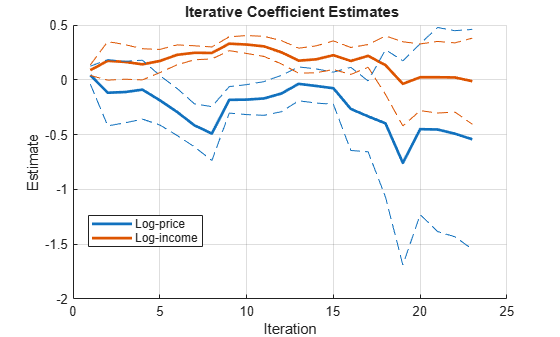Plot forward recursive-regression coefficient estimates using a rolling window 1/3 of the sample size.

window = ceil(T*1/3); recreg(X,y,Window=window,Plot="combined",PlotVars=plotvars, ... VarNames=varnames);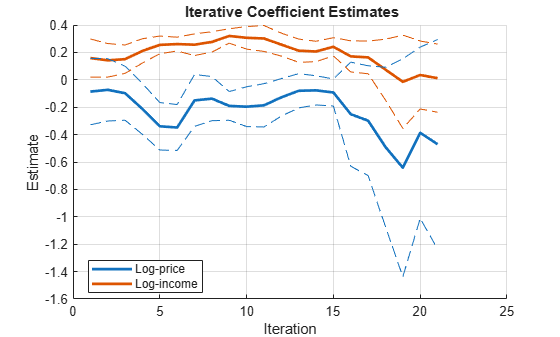Plot forward recursive-regression coefficient estimates using a rolling window of size 1/2 of the sample size.

window = ceil(T*1/2); recreg(X,y,Window=window,Plot="combined",PlotVars=plotvars, ... VarNames=varnames);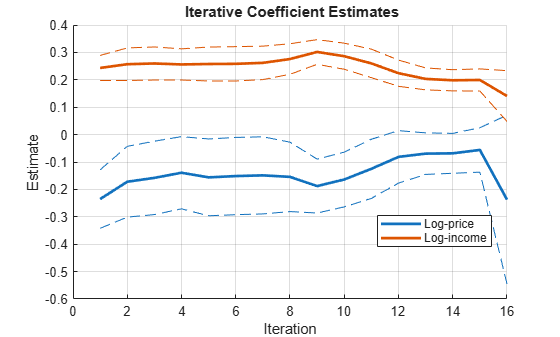As the window size increases, the lines show less volatility, but the coefficients do exhibit instability.

Apply recursive regressions using nested windows to look for instability in an explanatory model of real GNP for a period spanning World War II.

load Data_NelsonPlosser

The time series in the data set contain annual, macroeconomic measurements from 1860 to 1970. For more details, a list of variables, and descriptions, enter Description in the command line.

Several series have missing data. Focus the sample to measurements from 1915 to 1970. Identify the index corresponding to 1945, the end of WWII, to use as a breakpoint for the test.

span = (1915 <= dates) & (dates <= 1970); bp = find(dates(span) == 1945);

Consider the multiple linear regression model

${\text{GNPR}}_{t}={\beta }_{0}+{\beta }_{1}{\text{IPI}}_{t}+{\beta }_{2}{\text{E}}_{t}+{\beta }_{3}{\text{WR}}_{t}.$

Collect the model variables into a tabular array. Position the predictors in the first three columns and the response in the last column. Compute the number of coefficients in the model.

Tbl = DataTable(span,[4,5,10,1]); numCoeff = height(Tbl); 

Estimate the coefficients using recursive regressions, and return separate plots for the iterative estimates. Identify the iteration corresponding to the end of the war.

recreg(Tbl);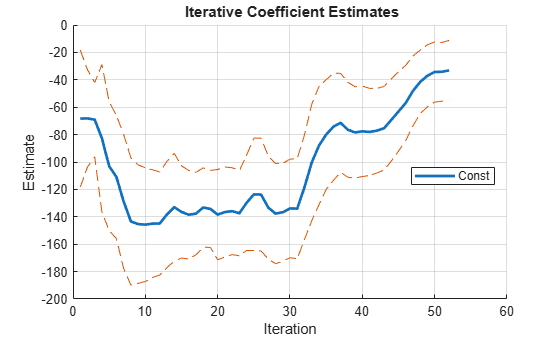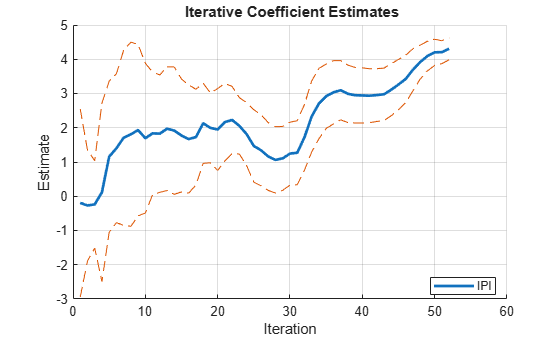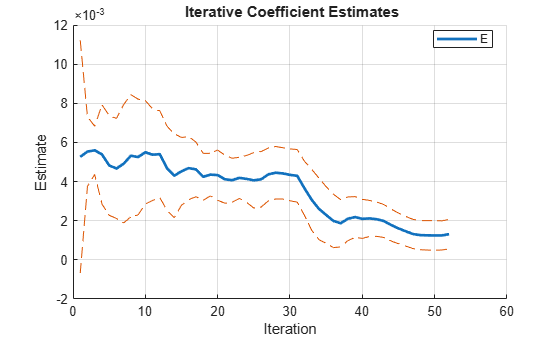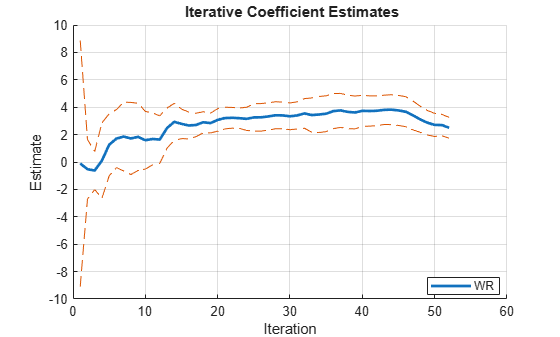bpIter = bp - numCoeff
bpIter = -25 

By default, recreg forms the subsamples using nested windows. The end of the war (1945) occurs at the 27th iteration.

All coefficients show some initial, transient instability during the "burn-in" period (see Tip). The plot of WR seems stable since the line is relatively flat. However, the plots of E, IPI, and the intercept (Const) show instability, particularly just after iteration 27.

Return tables of iterative coefficient estimates and a table of standard errors.

[CoeffTbl,SeTbl] = recreg(Tbl)
CoeffTbl=4×52 table Iter1 Iter2 Iter3 Iter4 Iter5 Iter6 Iter7 Iter8 Iter9 Iter10 Iter11 Iter12 Iter13 Iter14 Iter15 Iter16 Iter17 Iter18 Iter19 Iter20 Iter21 Iter22 Iter23 Iter24 Iter25 Iter26 Iter27 Iter28 Iter29 Iter30 Iter31 Iter32 Iter33 Iter34 Iter35 Iter36 Iter37 Iter38 Iter39 Iter40 Iter41 Iter42 Iter43 Iter44 Iter45 Iter46 Iter47 Iter48 Iter49 Iter50 Iter51 Iter52 _________ _________ _________ _________ _________ _________ _________ _________ ________ _________ _________ _________ _________ _________ _________ _________ _________ _________ _________ _________ _________ _________ _________ _________ _________ _________ _________ _________ _________ _________ _________ _________ _________ _________ _________ _________ _________ _________ _________ ________ _________ _________ _________ _________ _________ _________ _________ ________ _________ _________ _________ _________ Const -68.313 -68.159 -69.09 -82.751 -103.08 -110.89 -128.78 -143.35 -145.34 -145.7 -144.99 -144.89 -138.22 -132.97 -136.44 -138.5 -137.67 -133.23 -134.23 -138.4 -136.51 -135.89 -137.4 -129.78 -123.62 -123.76 -133.27 -137.67 -136.66 -133.9 -134.03 -118.43 -100.44 -88.055 -79.962 -74.23 -71.441 -76.452 -78.424 -77.625 -78.071 -77.155 -75.387 -69.307 -63.127 -56.891 -48.067 -41.498 -37.059 -34.289 -34.159 -33.111 IPI -0.19396 -0.26934 -0.23743 0.1147 1.1563 1.4004 1.7091 1.8108 1.9295 1.6962 1.8353 1.8286 1.9712 1.9167 1.7732 1.6692 1.7286 2.1302 1.995 1.9489 2.1656 2.2272 2.0554 1.8136 1.4672 1.3415 1.1593 1.0618 1.1066 1.2453 1.2701 1.7212 2.3317 2.7073 2.9193 3.0347 3.0946 2.9913 2.9503 2.9431 2.9357 2.9536 2.9781 3.1112 3.2635 3.4263 3.6943 3.9185 4.0934 4.2018 4.208 4.3063 E 0.0052645 0.0055309 0.0055981 0.0053891 0.0048281 0.0046665 0.0049113 0.0053202 0.005254 0.0054972 0.0053744 0.0053981 0.0046767 0.0043011 0.0045254 0.0046878 0.0046215 0.0042438 0.0043549 0.0043299 0.0041189 0.0040688 0.0041893 0.0041366 0.0040636 0.0041145 0.0043661 0.0044527 0.0044182 0.0043419 0.0042948 0.0036566 0.0030707 0.0026066 0.0022932 0.0019903 0.0018696 0.0020991 0.0021872 0.002095 0.0021159 0.0020759 0.0019813 0.0017761 0.0016083 0.0014596 0.0013216 0.001264 0.0012525 0.0012474 0.0012488 0.0013136 WR -0.1097 -0.52635 -0.62228 0.10675 1.2592 1.7072 1.8568 1.7174 1.8357 1.591 1.6799 1.6407 2.4802 2.9305 2.7864 2.664 2.7035 2.8985 2.8403 3.0737 3.208 3.2268 3.1978 3.1503 3.2559 3.2607 3.3254 3.4107 3.4009 3.3355 3.3988 3.5459 3.427 3.4651 3.5263 3.7157 3.7646 3.6554 3.616 3.7333 3.7218 3.7393 3.8059 3.8214 3.7642 3.6691 3.4004 3.1159 2.866 2.7074 2.6967 2.4962 
SeTbl=4×52 table Iter1 Iter2 Iter3 Iter4 Iter5 Iter6 Iter7 Iter8 Iter9 Iter10 Iter11 Iter12 Iter13 Iter14 Iter15 Iter16 Iter17 Iter18 Iter19 Iter20 Iter21 Iter22 Iter23 Iter24 Iter25 Iter26 Iter27 Iter28 Iter29 Iter30 Iter31 Iter32 Iter33 Iter34 Iter35 Iter36 Iter37 Iter38 Iter39 Iter40 Iter41 Iter42 Iter43 Iter44 Iter45 Iter46 Iter47 Iter48 Iter49 Iter50 Iter51 Iter52 _________ __________ __________ ________ _________ _________ _________ _______ _________ _________ _________ _________ ________ _________ __________ __________ __________ __________ __________ __________ __________ __________ __________ __________ _________ __________ __________ __________ __________ __________ __________ __________ __________ __________ __________ __________ __________ __________ _________ __________ __________ ________ __________ ________ ________ __________ __________ __________ __________ __________ __________ __________ Const 25.046 17.761 13.542 26.937 23.487 22.49 24.476 23.21 21.624 20.733 19.635 18.804 19.415 19.645 16.837 16.147 15.053 14.482 14.056 16.46 16.437 15.861 15.51 17.403 20.502 20.579 18.795 18.287 17.856 17.98 18.278 19.066 21.269 21.564 20.02 19.564 18.059 17.337 16.675 16.51 15.886 15.449 15.237 15.021 14.431 13.733 12.725 11.6 11.285 10.99 10.724 10.927 IPI 1.372 0.8095 0.63806 1.3034 1.1015 1.0866 1.2787 1.3444 1.2505 1.0933 0.89532 0.85677 0.9022 0.93004 0.82359 0.78861 0.69814 0.5849 0.50843 0.59812 0.56616 0.48889 0.41153 0.45871 0.52933 0.51865 0.49419 0.48475 0.46515 0.45985 0.46714 0.47995 0.51801 0.5138 0.46896 0.46184 0.4317 0.41892 0.40603 0.40296 0.39324 0.38492 0.38225 0.3798 0.36581 0.34681 0.30661 0.24728 0.21661 0.19106 0.16727 0.16107 E 0.0029773 0.00088806 0.00061649 0.001268 0.0012745 0.0012768 0.0015111 0.00156 0.0014781 0.0013213 0.0011703 0.0011097 0.001074 0.0010721 0.00086491 0.00080439 0.00069756 0.00059868 0.00054492 0.00064127 0.00061363 0.00056167 0.00052574 0.00059848 0.0007086 0.00070974 0.00067556 0.00067026 0.00065521 0.00066166 0.00067179 0.00069178 0.00077754 0.00078678 0.00072299 0.00068182 0.00060376 0.00055766 0.0005213 0.00049814 0.00046066 0.000438 0.00042075 0.000408 0.000392 0.00037819 0.00037353 0.00037249 0.00037624 0.00037665 0.00037242 0.00037821 WR 4.4923 1.0841 0.69505 1.3781 1.1311 1.0576 1.2544 1.3167 1.2237 1.0535 0.94646 0.86648 0.73106 0.67509 0.53986 0.48929 0.42646 0.38471 0.35942 0.41265 0.39531 0.3785 0.37151 0.42274 0.49945 0.50132 0.50008 0.49299 0.48526 0.4894 0.49548 0.54059 0.62494 0.65764 0.65499 0.63966 0.62063 0.61069 0.59915 0.56739 0.55232 0.54357 0.53533 0.54476 0.54798 0.54741 0.52804 0.47669 0.44754 0.4222 0.38934 0.38022 

If a linear regression model violates classical linear model assumptions, then OLS coefficient standard errors are incorrect. However, recreg has options to estimate coefficients and standard errors that are robust to heteroscedastic or autocorrelated innovations.

Simulate a series from this piecewise regression model with AR(1) errors whose regression coefficient changes at time 51.

$\left\{\begin{array}{c}\begin{array}{l}{y}_{t}=5+3{x}_{t}+{u}_{t}\\ {u}_{t}=0.6{u}_{t-1}+{\epsilon }_{t}\end{array};t=1,...,50\\ \begin{array}{l}{y}_{t}=5-{x}_{t}+{u}_{t}\\ {u}_{t}=0.6{u}_{t-1}+{\epsilon }_{t}\end{array};t=51,...,100.\end{array}$

${\epsilon }_{t}$ is a series of Gaussian innovations with mean 0 and standard deviation 0.5. ${x}_{t}$ is Gaussian with mean 1 and standard deviation 0.25.

rng(1); % For reproducibility T = 100; muX = 1; sigmaX = 0.25; x = sigmaX*randn(T,1) + muX; ar = 0.6; sigma = 0.5; c = 5; b = [3 -1]; y = zeros(T,1); Mdl1 = regARIMA(AR=ar,Variance=sigma,Intercept=c,Beta=b(1)); y(1:T/2) = simulate(Mdl1,T/2,X=x(1:T/2)); Mdl2 = regARIMA(AR=ar,Variance=sigma,Intercept=c,Beta=b(2)); y((T/2 + 1):T) = simulate(Mdl2,T/2,X=x((T/2 + 1):T));

Estimate recursive regression coefficients using OLS.

[CoeffOLS,SEOLS] = recreg(x,y,Plot="separate");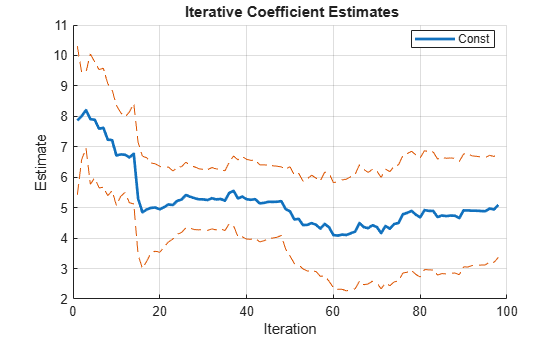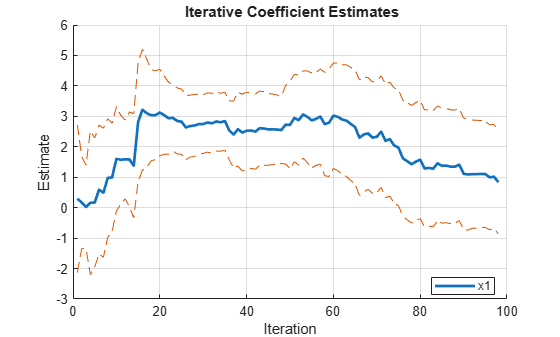After transient effects, 5 is within the confidence bounds of the intercept estimates. There is an insignificant but persistent shock at iteration 50. The coefficient estimates show the structural change after iteration 60.

To account for autocorrelated innovations, estimate recursive regression coefficients using OLS, but with Newey-West robust standard errors. For estimating the HAC standard errors, use the quadratic-spectral weighting scheme.

hacOptions.Weights = "QS"
hacOptions = struct with fields: Weights: "QS" 
[CoeffNW,SENW] = recreg(x,y,Estimator="hac",Options=hacOptions, ... Plot="separate");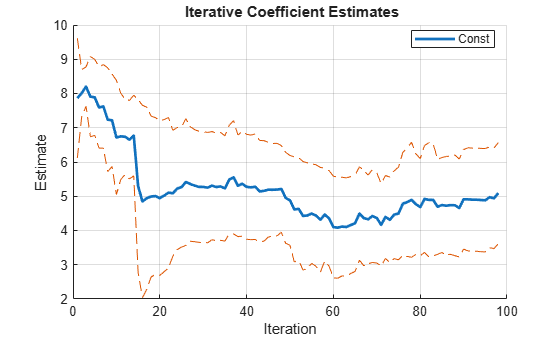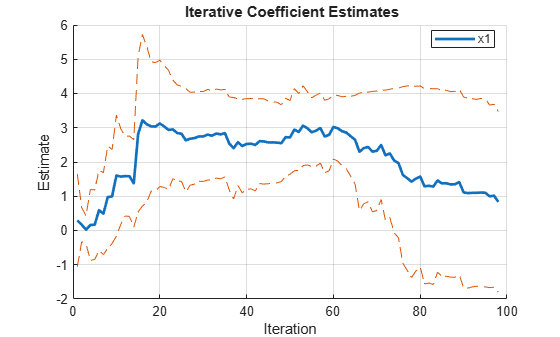The HAC coefficient estimates are the same as the OLS estimates. The confidence bounds are slightly different because the standard error estimators are different.

## Input Arguments

collapse all

Predictor data X for the multiple linear regression model, specified as a numObs-by-numPreds numeric matrix.

Each row represents one of the numObs observations and each column represents one of the numPreds predictor variables.

Data Types: double

Response data y for the multiple linear regression model, specified as a numObs-by-1 numeric vector. Rows of y and X correspond.

Data Types: double

Combined predictor and response data for the multiple linear regression model, specified as a table or timetable with numObs rows. Each row of Tbl is an observation.

The test regresses the response variable, which is the last variable in Tbl, on the predictor variables, which are all other variables in Tbl. To select a different response variable for the regression, use the ResponseVariable name-value argument. To select different predictor variables, use the PredictorNames name-value argument to select numPreds predictors.

Axes on which to plot, specified as a vector of Axes objects with length equal to the number of plots specified by the Plot and PlotVars name-value pair arguments.

By default, recreg creates a separate figure for each plot.

Note

NaNs in X, y, or Tbl indicate missing values, and recreg removes observations containing at least one NaN. That is, to remove NaNs in X or y, recreg merges the variables [X y], and then it uses list-wise deletion to remove any row that contains at least one NaN. recreg also removes any row of Tbl containing at least one NaN. Removing NaNs in the data reduces the sample size and can create irregular time series.

### Name-Value Arguments

Specify optional pairs of arguments as Name1=Value1,...,NameN=ValueN, where Name is the argument name and Value is the corresponding value. Name-value arguments must appear after other arguments, but the order of the pairs does not matter.

Before R2021a, use commas to separate each name and value, and enclose Name in quotes.

Example: recreg(Tbl,ResponseVariable="GDP",Intercept=false,Estimator="fgls") excludes an intercept term from the regression model, in which the response variable is the variable GDP in the table Tbl, and uses FGLS to estimate coefficients and standard errors.

Flag to include a model intercept, specified as a value in this table.

ValueDescription
truerecreg includes an intercept term in the regression model. numCoeffs = numPreds + 1.
falserecreg does not include an intercept when fitting the regression model. numCoeffs = numPreds.

Example: Intercept=false

Data Types: logical

Window length, specified as a numeric scalar.

• To compute estimates using nested windows, do not specify Window. In this case, recreg begins with the first numCoeffs + 1 observations, and then adds one observation at each iteration. The number of iterations is numIter = numObsnumCoeffs.

• To compute estimates using a rolling window, specify a window length. In this case, recreg shifts by one observation at each iteration. Window must be at least numCoeffs + 1 and at most numObs. The number of iterations is numIter = numObsWindow + 1.

Example: Window=10

Data Types: double

Estimation method, specified as a value in this table.

ValueDescription
"ols"

Ordinary least squares

"hac"

Heteroscedasticity and autocorrelation consistent (HAC) standard errors

"fgls"

Feasible generalized least squares coefficients and standard errors

Values "hac" and "fgls" call hac and fgls, respectively, with optional name-value arguments specified by Options.

Example: Estimator="fgls"

Data Types: char | string

hac and fgls optional name-value argument names and corresponding values, specified as a structure scalar.

Use Options to set any name-value argument except VarNames, Intercept, Display, Plot, ResponseVariable, and PredictorVariables. For these options, see corresponding recreg name-value arguments.

By default, recreg calls hac or fgls using defaults. If Estimator="ols", recreg ignores Options.

Example: Options=struct("ARLags",2) includes two lags in the AR innovations model for FGLS estimators.

Data Types: struct

Iteration direction, specified as a value in this table.

ValueDescription
"forward"

Forward recursions move the window of observations from the beginning of the data to the end.

"backward"

Backward recursions first reverse the order of observations, and then implement forward recursions.

Example: Direction="backward"

Data Types: char | string

Coefficient estimate plot control, specified as a value in this table. Plots show iterative coefficient estimates with ±2 standard error bands.

ValueDescription
"separate"recreg produces separate figures for each coefficient.
"combined"recreg combines all plots in a single set of axes.
"off"recreg turns off all plotting.

The defaults are:

• "off" when recreg returns output arguments

• "separate" otherwise

Example: Plot=off

Data Types: char | string

Flags for which coefficients to plot, specified as a logical vector of length numCoeffs. The first element corresponds to Intercept, if present, followed by indicators for each of the numPred predictors in X or Tbl. The default is true(numCoeffs,1) to plot all coefficients.

Example: PlotVars=[false true true false] plots the second and third coefficients of four coefficients.

Data Types: logical

Variable names for plotted coefficients, specified as a string vector or cell vector of strings of a length numCoeffs:

• If Intercept=true, VarNames(1) is the name of the intercept (for example 'Const') and VarNames(j + 1) specifies the name to use for variable X(:,j) or PredictorVariables(j).

• If Intercept=false, VarNames(j) specifies the name to use for variable X(:,j) or PredictorVariables(j).

The default is one of the following alternatives prepended by 'Const' when an intercept is present in the model:

• {'x1','x2',...} when you supply inputs X and y

• Tbl.Properties.VariableNames when you supply input table or timetable Tbl

Example: VarNames=["Const" "AGE" "BBD"]

Data Types: char | cell | string

Variable in Tbl to use for response, specified as a string vector or cell vector of character vectors containing variable names in Tbl.Properties.VariableNames, or an integer or logical vector representing the indices of names. The selected variables must be numeric.

recreg uses the same specified response variable for all tests.

Example: ResponseVariable="GDP"

Example: ResponseVariable=[true false false false] or ResponseVariable=1 selects the first table variable as the response.

Data Types: double | logical | char | cell | string

Variables in Tbl to use for the predictors, specified as a string vector or cell vector of character vectors containing variable names in Tbl.Properties.VariableNames, or an integer or logical vector representing the indices of names. The selected variables must be numeric.

recreg uses the same specified predictors for all tests.

By default, recreg uses all variables in Tbl that are not specified by the ResponseVariable name-value argument.

Example: PredictorVariables=["UN" "CPI"]

Example: PredictorVariables=[false true true false] or DataVariables=[2 3] selects the second and third table variables.

Data Types: double | logical | char | cell | string

## Output Arguments

collapse all

Coefficient estimates of each subsample regression, returned as a numCoeffs-by-numIter numeric matrix. recreg returns Coeff when you supply the inputs X and y.

The first row contains the intercept, if present, followed by rows for predictor coefficients in the column order of X or Tbl. Window determines numIter, the number of columns.

Standard error estimates of each subsample regression, returned as a numCoeffs-by-numIter numeric matrix. recreg returns SE when you supply the inputs X and y.

Row order and number of columns correspond to Coeff.

Coefficient estimates of each subsample regression returned as a numCoeffs-by-numIter table. recreg returns CoeffTbl when you supply the input Tbl.

For i = 1,…,numCoeffs, row i of CoeffTbl contains estimates of coefficient i in the regression model and it has label VarNames(i). Variable j contains the estimates of iteration j and it has label Iterj.

Standard error estimates of each subsample regression, returned as a numCoeffs-by-numCoeffs table containing the coefficient covariance matrix estimate EstCoeffCov. recreg returns SETbl when you supply the input Tbl.

For i = 1,…,numCoeffs, row i of SETbl contains the standard error estimates of coefficient i in the regression model and it has label VarNames(i). Variable j contains the estimates of iteration j and it has label Iterj.

Handles to plotted graphics objects, returned as a vector of graphics objects. coeffPlots contains unique plot identifiers, which you can use to query or modify properties of the plot.

coeffPlots is not available if the value of the Plot name-value argument is "off".

## Tips

Plots of nested-window estimates typically show volatility during a “burn-in” period, in which the number of subsample observations is only slightly larger than the number of coefficients in the model. After this period, any further volatility is evidence of coefficient instability. Sudden changes in coefficient values can indicate a structural change, and sustained changes can indicate model misspecification. For structural change tests, see cusumtest and chowtest.

 Enders, Walter. Applied Econometric Time Series. Hoboken, NJ: John Wiley & Sons, Inc., 1995.

 Johnston, J. and J. DiNardo. Econometric Methods. New York: McGraw Hill, 1997.